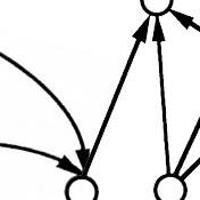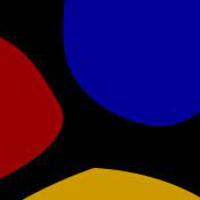## Scanning QR Codes

Recognize QR Codes in images from scratch. We'll do all the bit math to figure out the location markers and then read data from the black/white array.

## Locating the QR code

Series: Scanning QR Codes:

1. Introduction
2. Locating the QR code
3. Verifying the finder patterns
4. Extracting bits from the QR code

In this and the next part, we'll look at finding the three "finder" patterns and detecting the QR code. Once we've done this, we will have an estimate of the orientation of the code and also the size of each block.

## The main function

Let’s get started with the project. Create a file named main.cpp and add the following code to it:

```#include <iostream>
#include <opencv2/opencv.hpp>

using namespace std;
using namespace cv;

int main(int argc, char* argv[])
{
```

Now, for this project, we’ll make a class that tries to identify a QR code in any image you give to it. We’ll name it, hmm, qrReader. Till now, we’ve just included the basic stuff – namespaces, opencv headers, vector, etc.

Next, we try to get a hold of the image passed to our program through command line arguments. We also convert it into a greyscale image and threshold it so that we're only left with black or white pixels.

```    if(argc == 1) {
cout << "Usage: " << argv << " <image>" << endl;
exit(0);
}

Mat imgBW;
cvtColor(img, imgBW, CV_BGR2GRAY);
```

Now, you do the actual detection. If a code was actually found, draw it!

```    qrReader reader = qrReader();
if(found) {
}
```

Finally, show whatever we got, wait until a key is pressed and quit. This completes the framework for the next couple of parts.

```    imshow("image", img);
waitKey(0);

return 0;
}
```

Lets create the basic declaration of the class. Here's what my header file `qrReader.h` looks like:

```#pragma once
#include <opencv2/opencv.hpp>
using namespace std;
using namespace cv;

public:
bool find(const Mat& img);
void drawFinders(Mat& img);

private:
bool checkRatio(int* stateCount);
bool handlePossibleCenter(const Mat& img, int* stateCount, int row, int col);
bool crossCheckDiagonal(const Mat &img, float centerRow, float centerCol, int maxCount, int stateCountTotal);
float crossCheckVertical(const Mat& img, int startRow, int centerCol, int stateCount, int stateCountTotal);
float crossCheckHorizontal(const Mat& img, int centerRow, int startCol, int stateCount, int stateCountTotal);

inline float centerFromEnd(int* stateCount, int end) {
return (float)(end-stateCount-stateCount)-(float)stateCount/2.0f;
}

vector<Point2f> possibleCenters;
vector<float> estimatedModuleSize;
};
```

You've already looked at the `find` and `drawFinders` methods. They do the actual detection and rendering the detection on the original image. In this post, we'll be working on them along with the `checkRatio` method. We'll look at the `handlePossibleCenter and`crossCheck` methods in the next post.

## The state logic

The finder patterns have the 1:1:3:1:1 ratio. This is the key to detecting them. Here’s how it works: The code goes through every row and keeps a track of the number of white/black pixels it encounters. It also keeps a track of the order in which they’re found. Whenever it finds something in the expected order, it assumes it found finder pattern. The `checkRatio` function helps with this.

The code can be in 5 states.

• State 0: Inside black pixels / looking for the first black pixel
• State 1: Inside white pixels
• State 2: Inside black pixels
• State 3: Inside white pixels
• State 4: Inside black pixels

So how do you move from one state to the other? You might recall a finite state automata from your CS class. I’ll describe something similar to it. We’ll add more checks to ensure it actually is a finder patter in the next post.

### The implementation

Create a new file: qrreader.cpp. We’ll include the header file and create a function that does the detection:

```#include "qrReader.h"

{
```

This function takes in an image to scan and returns true if it found a QR code. You can use the class functions to access information about most recent QR code (location, orientation, etc).

Earlier, I mentioned the the detector goes through each line. You could do that, but it turns out that scanning every third line makes it work quite as well too. So I’ve introduced a new variable `skipRows` that you can tweak. We also clear any past detections.

```    possibleCenters.clear();
estimatedModuleSize.clear();

int skipRows = 3;
```

Now, we initialize an array that maintains the state data and create a variable that keeps track of the current state. We also create a loop that iterates through the image.

```    int stateCount = {0};
int currentState = 0;
for(int row=skipRows-1; row<img.rows; row+=skipRows)
{
```

Now, we initialize `stateCount` to zero and set the current state to zero. This is so you can check if every row has the finder pattern. You need to erase state data from the previous rows.

```        stateCount = 0;
stateCount = 0;
stateCount = 0;
stateCount = 0;
stateCount = 0;
currentState = 0;
```

Next, get a pointer to the current row’s raw pixels and start iterating across it:

```        const uchar* ptr = img.ptr<uchar>(row);
for(int col=0; col<img.cols; col++)
{
```

Now, we check if we’re at a black pixel. If we are and the state is one of being inside a white (state 1 and state 3), we move to the next state. And for either case (being inside white or black), we increment the stateCount of the ‘current’ state:

```            if(ptr[col]<128)
{
// We're at a black pixel
if((currentState & 0x1)==1)
{
// We were counting white pixels
// So change the state now

// W->B transition
currentState++;
}

// Works for boths W->B and B->B
stateCount[currentState]++;
}
```

Now, if we’re at a white pixel:

```            else
{
```

If we were counting white pixels, simply increment the stateCount of the correct state:

```                // We got to a white pixel...
if((currentState & 0x1)==1)
{
// W->W change
stateCount[currentState]++;
}
```

If not, we need to check some conditions. This is slightly tricky:

```                else
{
// ...but, we were counting black pixels
if(currentState==4)
{
```

So what we do is, check if the ratio is what we expected – 1:1:3:1:1

```                        // We found the 'white' area AFTER the finder patter
// Do processing for it here
if(checkRatio(stateCount))
{
```

And you know that you’re at a possible finder pattern. More checks need to be done to ensure this is an actual finder pattern. We’ll do that in the next post. So, for now, I’ll just leave a comment:

```                            // This is where we do some more checks
}
```

We could also check if we found all the three finder patterns. If we did, return a true. But we’ll do that in the next part. But, if the ratio isn’t right, we need to do the switch I mentioned earlier:

```                        else
{
currentState = 3;
stateCount = stateCount;
stateCount = stateCount;
stateCount = stateCount;
stateCount = 1;
stateCount = 0;
continue;
}
```

And other than that, here’s what we gotta do:

```                        currentState = 0;
stateCount = 0;
stateCount = 0;
stateCount = 0;
stateCount = 0;
stateCount = 0;
}
else
{
// We still haven't go 'out' of the finder pattern yet
// So increment the state
// B->W transition
currentState++;
stateCount[currentState]++;
}
```

Now, close all the loops and return if we found any centers:

```                }
}
}
}
return (possibleCenters.size()>0);
}
```

Now for how we check the ratio:

```bool qrReader::checkRatio(int stateCount[])
{
int totalFinderSize = 0;
for(int i=0; i<5; i++)
{
int count = stateCount[i];
totalFinderSize += count;
if(count==0)
return false;
}

if(totalFinderSize<7)
return false;

// Calculate the size of one module
int moduleSize = ceil(totalFinderSize / 7.0);
int maxVariance = moduleSize/2;

bool retVal= ((abs(moduleSize - (stateCount)) < maxVariance) &&
(abs(moduleSize - (stateCount)) < maxVariance) &&
(abs(3*moduleSize - (stateCount)) < 3*maxVariance) &&
(abs(moduleSize - (stateCount)) < maxVariance) &&
(abs(moduleSize - (stateCount)) < maxVariance));

return retVal;
}
```

Now this is interesting. We calculate the total ‘width’ of the pattern (`totalFinderCount`) in terms of pixels. If we ever encounter a ‘count’ that’s zero (meaning a white or black patch that didn’t exist), we’re sure that this isn’t a valid finder pattern. So just return a false. If the width of the pattern is less than seven, we’re sure it can’t be a valid pattern either.

Then we calculate the approximate module size based on our current observations. This module size can be quite off the actual module size (because you can tilted patterns will make the module size 'appear' larger than they actually are). So we need to tolerate quite a big variation range. For this function, it is half of the approximate module (maxVariance).

So the difference between the approximate module size and actual counts (stateCount) should be less than this maximum variance. Since the middle area is made up of three modules – it needs to be withing thrice this maximum variance. If it is, there’s a good chance this might be a finder pattern.

We’ll add more checks in the next post and actually render the finder patterns.

## More in the series

This tutorial is part of a series called Scanning QR Codes:

1. Introduction
2. Locating the QR code
3. Verifying the finder patterns
4. Extracting bits from the QR code

#### Related posts#### 7 unique neural network architectures#### Connected Component Labelling

Utkarsh Sinha created AI Shack in 2010 and has since been working on computer vision and related fields. He is currently at Microsoft working on computer vision.